# The Tridiminished Icosahedron

The tridiminished icosahedron is one of the Johnson solids. It is bounded by 5 regular triangles and 3 regular pentagons, for a total of 8 faces, 15 edges, and 9 vertices. It may be constructed by removing 3 vertices from the regular icosahedron such that 3 pentagonal faces are formed. It is the 63rd polyhedron in Norman Johnson's list, and thus bears the label J63.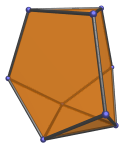There are three different kinds of triangular faces: the top triangle, which is surrounded by three pentagonal faces; the bottom triangle, which is surrounded by three triangular faces; and three lateral triangles surrounding the bottom triangle.

Due to the non-equivalence of its vertices, the tridiminished icosahedron only has a single axis of symmetry: a 3-fold symmetry around the line passing through the top and bottom triangles.

The top triangle can be augmented with a tetrahedron to form the augmented tridiminished icosahedron (J64), another Johnson solid.

The tridiminished icosahedron is the vertex figure of the snub 24-cell, and occurs as cells in the bi-icositetradiminished 600-cell.

## Projections

In order to be able to identify the tridiminished icosahedron in various projections of 4D objects, it is useful to know how it appears from various viewpoints. The following are some of the viewpoints that are commonly encountered:

Projection Envelope Description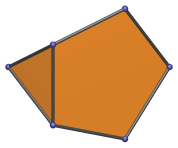Irregular pentagon

Projection parallel to an edge of the top triangle. This projection has the most number of coincident faces: two pentagonal faces project to the pentagonal area, the third pentagonal face to the upper left edge; the top triangle to the upper right edge; the bottom triangle to the lower left edge; two lateral triangles to the triangular area; the third lateral triangle to the bottom edge.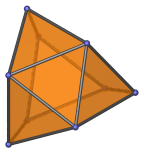Irregular hexagon

Projection centered on top and bottom triangles. This is the most symmetric projection of the tridiminished icosahedron.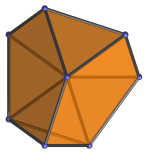Irregular heptagon

Projection centered on vertex surrounded by two pentagons and a lateral triangle.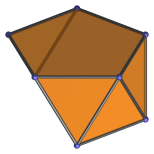Irregular pentagon

Projection centered on the edge between a pentagon and a lateral triangle. The bottom left edge of the projection envelope is the image of a pentagonal face.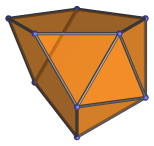Irregular hexagon

Projection centered on a lateral triangle.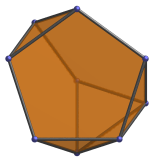Irregular octagon

Projection centered on a pentagonal face.

## Coordinates

The Cartesian coordinates of the tridiminished icosahedron are:

• (0, 1, φ)
• (0, ±1, −φ)
• ( 1, φ, 0)
• (±1, −φ, 0)
• ( φ, 0, 1)
• (−φ, 0, ±1)

where φ=(1+√5)/2 is the Golden Ratio.

These coordinates are obtained by deleting 3 vertices from the regular icosahedron such that 3 pentagonal faces are formed.

## Occurrences

The tridiminished icosahedron appears as cells in a good number of CRF polychora, generalizations of the Johnson solids to 4D, especially those related to the 600-cell family of uniform polychora. Its occurrences include (but are not limited to) the following polytopes:

Last updated 18 Jun 2019.